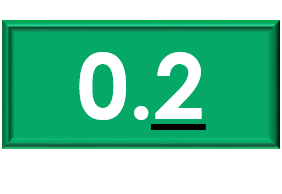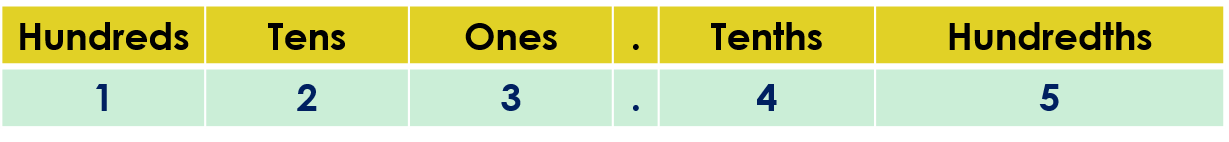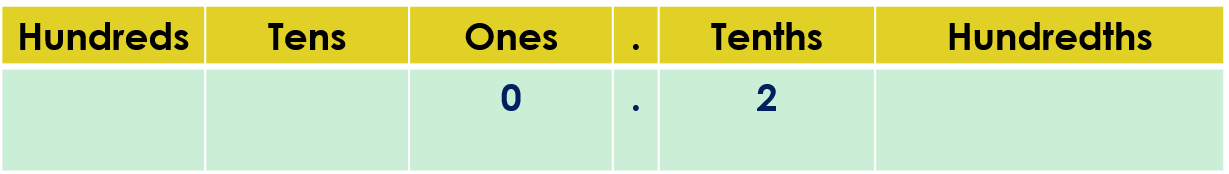1
visibility

What is the value of the underlined digit in the given number?• A

0

• B

0.2

• C

2

• D

20

The correct answer is "0.2".

Please Note:

Place value can be explained as the value represented by a digit in a number on the basis of its position in the number.

For example: Given the number 123.45.
Find the values of the digits 2 and 5.

But, the number 123.45 can be represented in the place value chart as:The value of the digit 2 is 2 tens or 20.
The value of the digit 5 is 5 hundredths or 0.05.

Solution:

Write the given number in the place value chart and identify the place of the underlined digit.To identify the place of the digit 2 in the number 0.2, we look for the position of 2 in the place value chart.

The digit 2 is in the tenths place.

So, the value of the digit 2 is 2 tenths or 0.2.